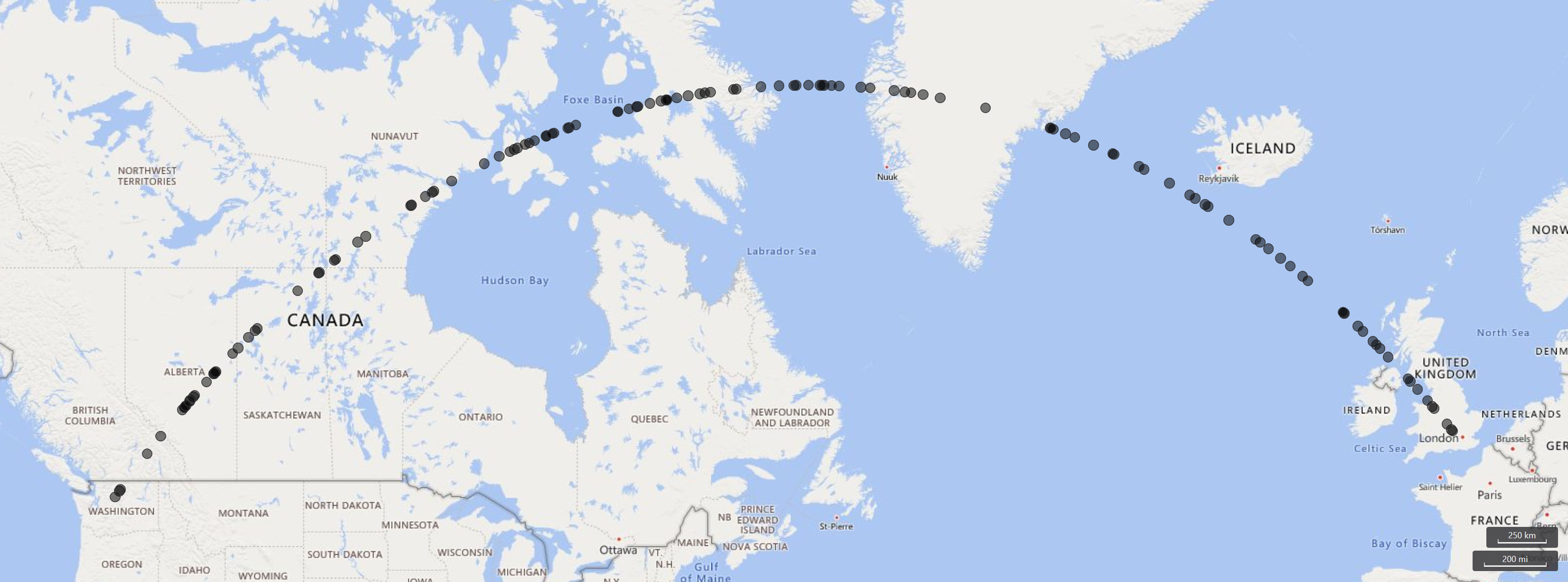# geo_distance_2points()

Calculates the shortest distance in meters between two geospatial coordinates on Earth.

## Syntax

`geo_distance_2points(`p1_longitude`,`p1_latitude`,`p2_longitude`,`p2_latitude`)`

## Parameters

Name Type Required Description
p1_longitude real The longitude value in degrees of the first geospatial coordinate. A valid value is in the range [-180, +180].
p1_latitude real The latitude value in degrees of the first geospatial coordinate. A valid value is in the range [-90, +90].
p2_longitude real The longitude value in degrees of the second geospatial coordinate. A valid value is in the range [-180, +180].
p2_latitude real The latitude value in degrees of the second geospatial coordinate. A valid value is in the range [-90, +90].

## Returns

The shortest distance, in meters, between two geographic locations on Earth. If the coordinates are invalid, the query will produce a null result.

Note

• The geospatial coordinates are interpreted as represented by the WGS-84 coordinate reference system.
• The geodetic datum used to measure distance on Earth is a sphere.

## Examples

The following example finds the shortest distance between Seattle and Los Angeles.``````print distance_in_meters = geo_distance_2points(-122.407628, 47.578557, -118.275287, 34.019056)
``````

Output

distance_in_meters
1546754.35197381

Here's an approximation of shortest path from Seattle to London. The line consists of coordinates along the LineString and within 500 meters from it.``````range i from 1 to 1000000 step 1
| project lng = rand() * real(-122), lat = rand() * 90
| where lng between(real(-122) .. 0) and lat between(47 .. 90)
| where geo_distance_point_to_line(lng,lat,dynamic({"type":"LineString","coordinates":[[-122,47],[0,51]]})) < 500
| render scatterchart with (kind=map)
``````

The following example finds all rows in which the shortest distance between two coordinates is between 1 meter and 11 meters.

``````StormEvents
| extend distance_1_to_11m = geo_distance_2points(BeginLon, BeginLat, EndLon, EndLat)
| where distance_1_to_11m between (1 .. 11)
| project distance_1_to_11m
``````

Output

distance_1_to_11m
10.5723100154958
7.92153588248414

The following example returns a null result because of the invalid coordinate input.

``````print distance = geo_distance_2points(300,1,1,1)
``````

Output

distance